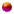﻿

# PRODUCT產品介紹

Torque Sensors（力矩感測器）

Rotating with slipring （迴轉型 - 滑環碳刷式）

Rotating contactless （迴轉型 - 非接觸式）

Rotating contactless （迴轉型雙量程 - 非接觸式）

Rotating contactless ... Screw Driving Systems （迴轉型 - 非接觸式）

Non-rotating (static) （非迴轉型 - 靜態）

Multi-Component Sensors（力矩 / 力量兩用型感測器）

Torque (static) / Force

Torque (rotary) / Force ... M-2371 (2 N.m / 100N....5 N.m / 500 N)Data Sheet Terms and Definitions

Software

 newton-meters (n-m) = [lb-in] x [0.113005] lb-in = [n-m] x [8.84918] newton-meters (n-m) = [kg-m] x [9.80839] kg-m = [n-m] x [0.101954] kilogram-meters (kg-m) = [lb-in] x [0.011521] lb-in = [kg-m] x [86.7962]

♦ Torque Sensors（力矩感測器）

Rotating - Slipring (迴轉型 - 滑環碳刷式)

Rotating contactless (迴轉型 - 非接觸式)

Rotating contactless (迴轉型雙量程 - 非接觸式)

Rotating contactless ... Screw Driving Systems (迴轉型 - 非接觸式)

Non-rotating (static) (非迴轉型 - 靜態)

Multi-Component Sensors（力矩 / 力量兩用型感測器）

Torque (static) / Force

Torque (rotary) / Force ... M-2371

(2 N.m / 100N....5 N.m / 500 N)

Data Sheet Terms and Definitions

For Torque Sensors

Software

 n-m = [lb-in] x [0.113005] n-m = [kg-m] x [9.80839] kg-m = [lb-in] x [0.011521] lb-in = [n-m] x [8.84918] kg-m = [n-m] x [0.101954] lb-in = [kg-m] x [86.7962]